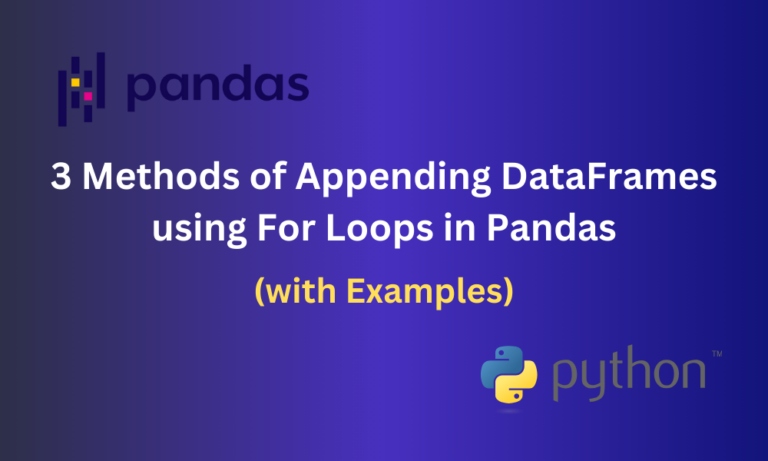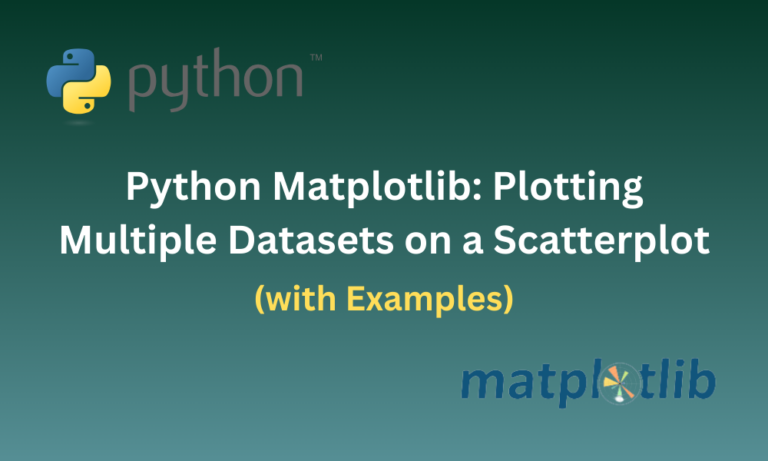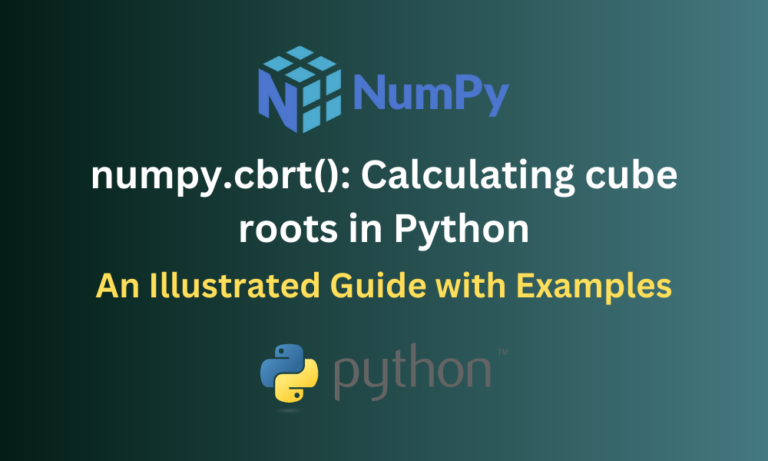## Appending Dataframes in Pandas (Multiple Examples)Pandas is a popular data manipulation library in Python that helps us work with various types of datasets. Pandas provides DataFrames, a two-dimensional tabular structure containing rows and columns that help us store said datasets. In many cases, we may…

## Plotting Multiple Datasets on a Scatterplot Using MatplotlibMatplotlib is a well-used tool by many developers when it comes to data visualization using graphical plots. It can also be used to generate plots for datasets created using Python libraries such as NumPy and Pandas. However, when it comes…

## numpy.cumprod() in Python: Calculating Cumulative Product in NumPyPython provides many libraries that can perform various functions in different fields. One such famous library is NumPy. NumPy provides various mathematical functions that help us perform computations on arrays, scalars and matrices. In this article, we’ll explore the numpy.cumprod()…

## numpy.cbrt() in Python: Calculating Cube Roots in NumPyNumPy is a famous and often-used Python library that provides various mathematical functions when it comes to performing operations on arrays. These functions make computations involving array elements easier and more efficient. In this article, we’ll look at one such…

## Working with JSON File in Python: A Comprehensive GuideJSON, which stands for JavaScript Object Notation is a widely used format for storing data in the form of key-value pairs, similar to an object in JavaScript. It is language-independent making it easier to exchange data throughout various programming languages.…